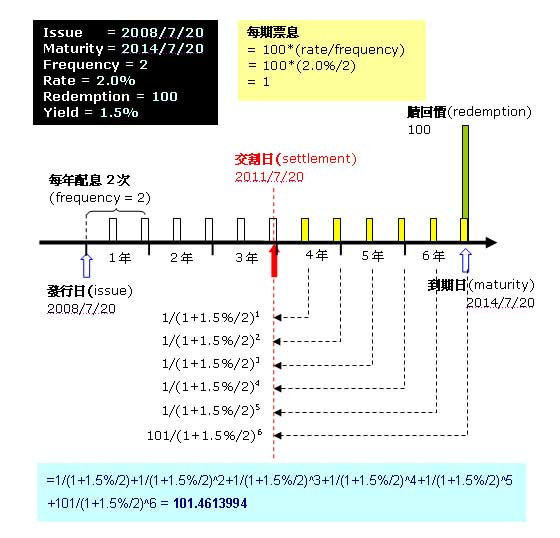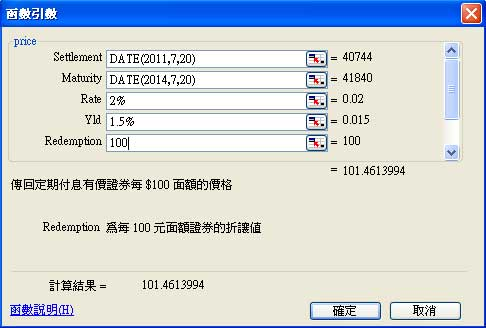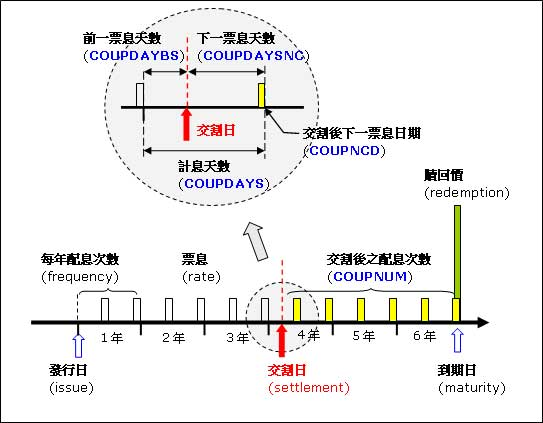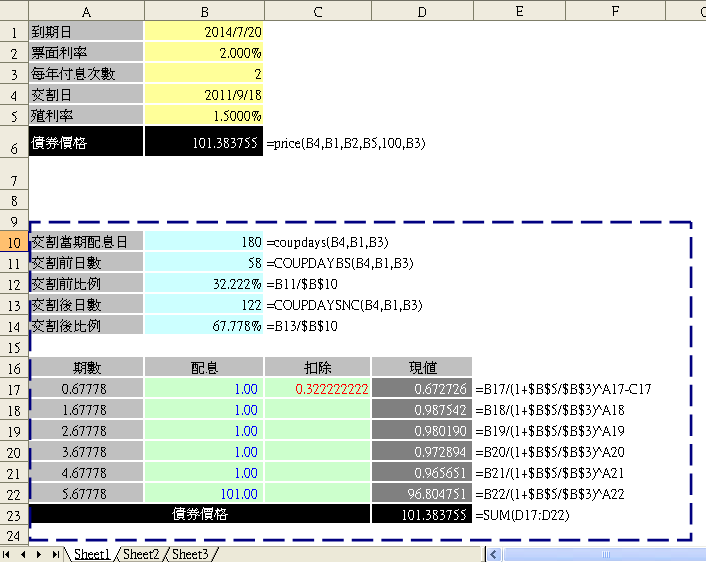﻿

# 債券價格(Price)和殖利率(Yield)• 第1期：1/(1+1.5%/2)1
• 第2期：1/(1+1.5%/2)2
• 第3期：1/(1+1.5%/2)3
• 第4期：1/(1+1.5%/2)4
• 第5期：1/(1+1.5%/2)5
• 第6期：101/(1+1.5%/2)6

Price = 1/(1+1.5%/2)1+1/(1+1.5%/2)2+1/(1+1.5%/2)3+1/(1+1.5%/2)4+1/(1+1.5%/2)5+101/(1+1.5%/2)6

=1/(1+1.5%/2)+1/(1+1.5%/2)^2+1/(1+1.5%/2)^3+1/(1+1.5%/2)^4+1/(1+1.5%/2)^5+101/(1+1.5%/2)^6
=101.4613994

# Excel PRICE函數

=price(DATE(2011,7,20),DATE(2014,7,20),2%,1.5%,100,2)
=101.4613994

1) 工具/增益集
2) 『分析工具箱』打勾
3) 按確定# 付息期間交割之債券價格## Excel檔案下載A10到D23範圍的儲存格，都只是為了說明PRICE函數是如何計算的明細表(藍色虛線框起來部份)。重點在交割日後第一期，因為不足一期，必須算出佔整期的比例，才有辦法將第1期的票息折現，第2期以後只要將前一期加一即可。計算方式是先算出交割日該期的計息日數有幾天(儲存格B10)，然後求出交割日到下一付息日的日數(儲存格B13)，兩者相除就可求出比例(儲存格B14)。由B14結果顯示，交割後到第一付息日為0.67778期，所以第1期的票息折現公式=1/(1+1.5%/2)0.67778，第2期=1/(1+1.5%/2)1.67778依此類推。

Price = 1/(1+1.5%/2)0.67778+1/(1+1.5%/2)1.67778+1/(1+1.5%/2)2.67778+1/(1+1.5%/2)3.67778+1/(1+1.5%/2)4.67778+101/(1+1.5%/2)5.67778-1*(58/180)

=1/(1+1.5%/2)^0.67778+1/(1+1.5%/2)^1.67778+1/(1+1.5%/2)^2.67778+1/(1+1.5%/2)^3.67778+1/(1+1.5%/2)^4.67778+101/(1+1.5%/2)^5.67778-1*(58/180) = 101.3837533

# 相關文章

﻿

■ 本網站內容儘可能精確完整，但不保證無誤。若做為投資依據，風險請自行斟酌 ，本網站不負賠償之責任。
■ 網站所有資料均為版權所有，非經書面允許請勿轉載或使用。